# Direction Sense Test

Direction is the measurement of position of one object with respect to another object and displacement is the minimum distance between the starting point and final point of an object.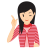Few Important things to Remember

In this section, we deal with the questions in which a successive follow-up of directions is formulated and the candidate is required to ascertain the final direction or the distance between two points.

Before moving ahead, let us familiarize with some facts/concepts.

Main Directions

There are four main directions viz. East, West, North and South as shown in the figure given below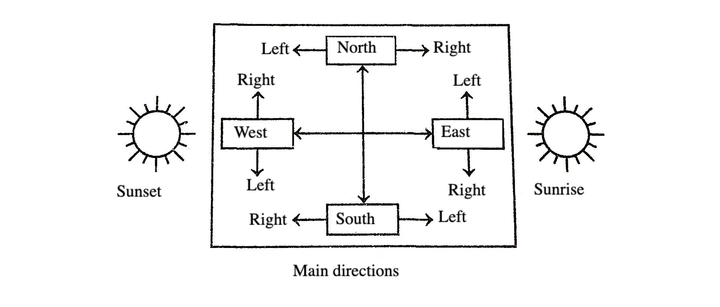Abbreviations for these directions are E (East), W (West), N (North) and S (South).

Cardinal Directions

A direction between two main directions is called cardinal direction or sub-direction, i.e. NE (North-East), NW (North-West), SE (South-East) and SW (South-West).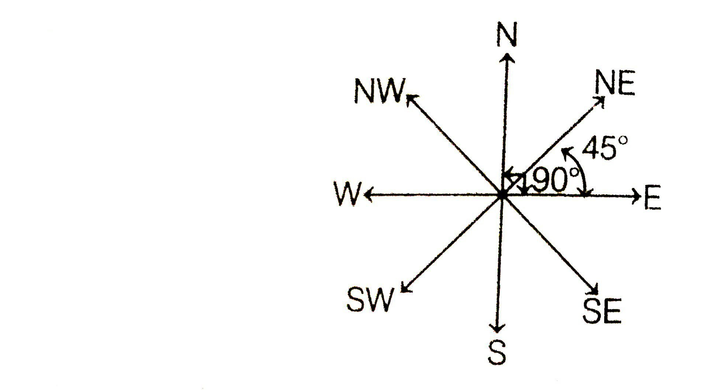Students are advised to use diagram as given here, for the purpose of sensing directions.

Angle formed between two main directions (i.e. North-East; North-West; South-East or South-West) is 90° and angle formed between a cardinal direction and main direction is 45°.

Angle of Movement

For solving questions based on angle of movement, it is necessary to know the rotations which are given below

(i) Movement towards the right is called clockwise (CW) movement.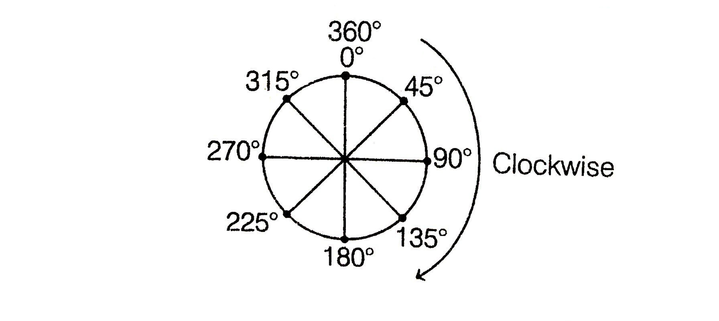(ii) Movement towards the left is called anti-clockwise (ACW) movement.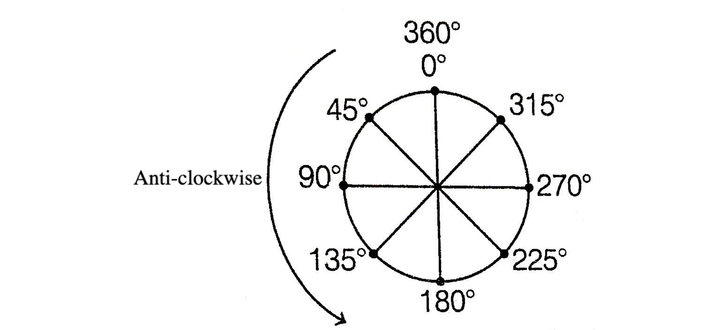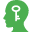Memory Add-ons

Shadow at the Time of Sunrise

In the morning, sunrise in the East, the shadow of any person or object falls in the West.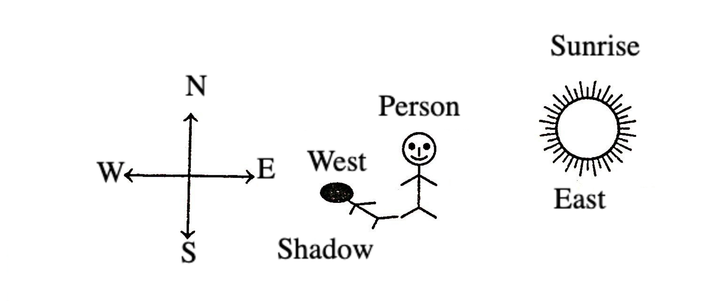Shadow at the Time of Sunset

In the evening, when the sunset in the West, the shadow of a person or an object falls in the East.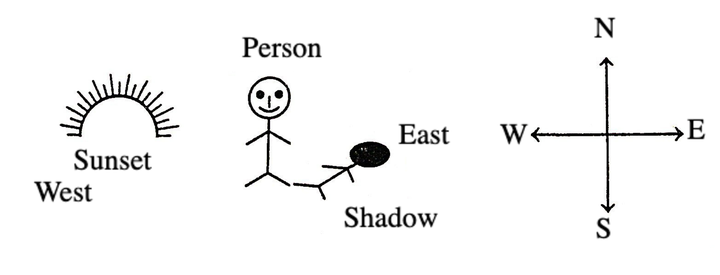Shortest Distance

If we draw a straight line from the initial point of the object to the final point, then length of this line is called shortest distance.

e.g. An object starts from point A and reaches to point C after going through point B.

Then, distance between initial and final position = AB + BC

But shortest distance between initial and final position = AC

To find the shortest distance between two points, it is necessary to know Pythagoras theorem.

Here, inAB = Perpendicular

BC = Base and

AC = Hypotenuse

Hence, the shortest distance between A and C

i.e. AC = AB2 + BC2

Example 1:  Ravi walks 8 km North-East and then 6 km South-East. Find the total distance as well as shortest distance between starting and end points.

A. 14 km, 12 km     B. 14 km, 10 km
C. 10 km, 8 km      D. 8 km, 6 km

Solution:  (B) According to the question,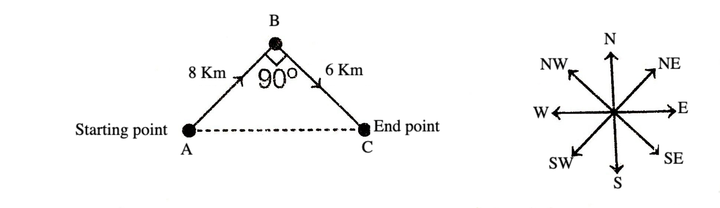Here, total distance = AB + BC

= 8 + 6 = 14 km

and shortest distance AC = AB2 + BC 2

= 82 + 6 2

= 64 + 36

= 100 = 10 km

## Types of Reasoning Questions on Direction Sense

There are four types of questions which are generally asked in various competitive examinations.

Type #1: Based on Final Direction

These question are based on finding the final direction of an object with respect to its starting point.

Example 1:  Suman walks 15 km towards North. She turns right and walks another 15 km. She turns right and walks another 15 km. In which direction is she from the starting point?

A. North     B. South     C. East    D. West

Solution:  (C) According to the question, the direction diagram of Suman is a given below.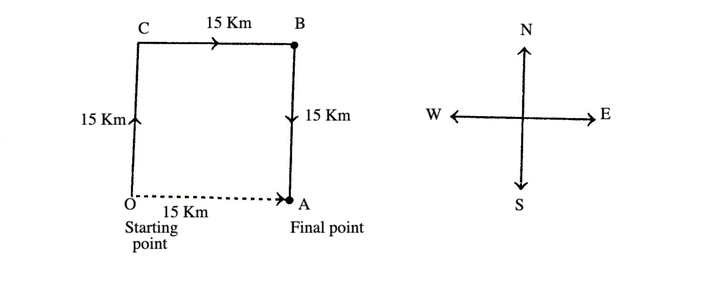Let O be the starting point and A be ending point of Suman. It is clear from the diagram that, she is in East direction from her starting point.

Example 2:  After starting from a point, a man walks 4 km towards West, then turning to his right he moves 4 km. After this, he again turns right and moves 4 km. Which choice given below indicates the correct direction in which he is from his starting point?

A. North                         B. East
C. South                        D. West

Solution:  (A) According to the question, the direction diagram of a man is as given below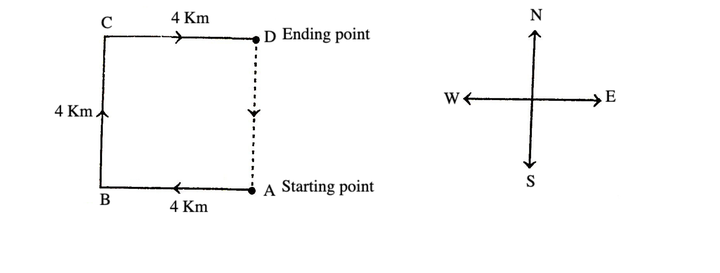Let A be the starting and D be the ending point of man. It is clear from diagram that, he is in North direction from his starting point.

Example 3:  If South-West becomes North, then what will North-East be?

A. North     B. South-East     C. South    D. East

Solution:  (C) The diagrammatic representation of direction is as shown below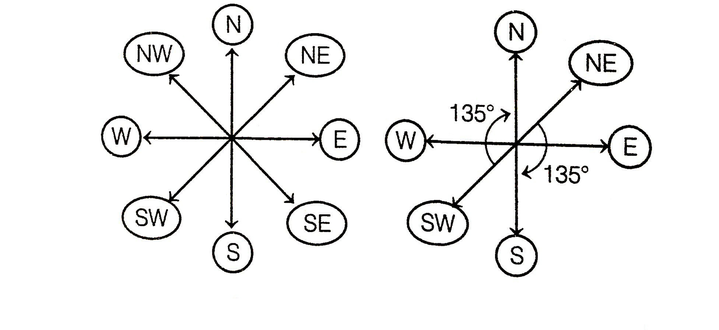Clearly, directions are moving 135° clockwise.
Hence, North-East will become South moving 135° clockwise

Example 4:  A clock is so placed that at 12 noon its minute hand points towards North-East. In which direction does its hour hand point at 1:30 pm?

A. North     B. South     C. East    D. West

Solution:  (C) In this question, the clock is placed, so that at 12 noon its minute hand point towards North-East.

We know that, minute and hour hand point in the same direction at 12 noon. Therefore, the clock will look some what like this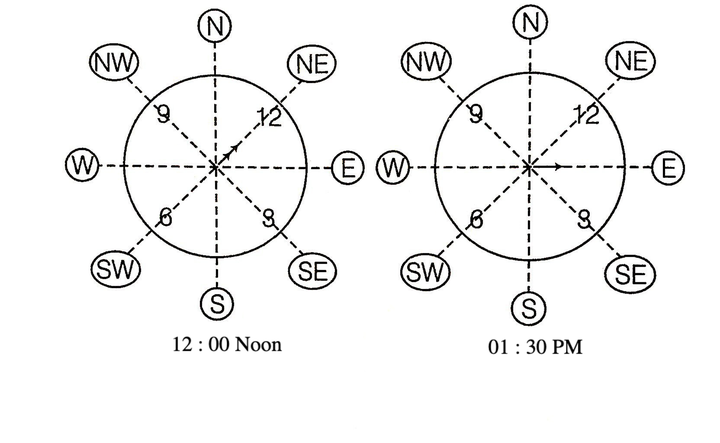At 1 : 30 pm, the hour hand will point in the East direction.

Example 5:  A girl is facing North. She turns 180° in the anti-clockwise direction and then 225° in the clockwise direction. Which direction is she facing now?

A. West                       B. North-East
C. South-West             D. East
E. North-West

Solution:  (B) The girl turns 180° in anti-clockwise direction and then 225° in the clockwise direction, which means she finally turns 45° in clockwise direction.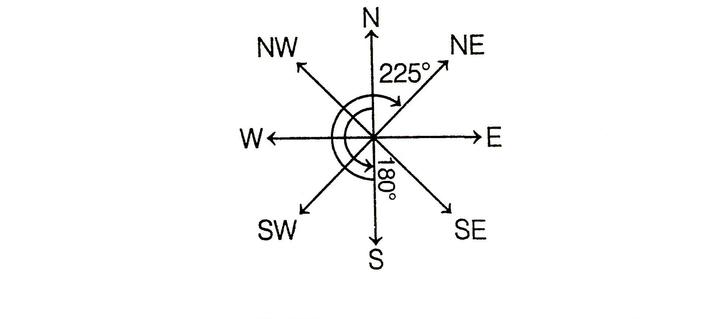Initially she was facing North. So, now she is facing North-East direction.

Example 6:  A person walks towards his house at 8:00 am and observes his shadow to his right. In which direction he is walking?

A. North     B. South     C. East    D. west

Solution:  (B) At 8:00 am the Sun is in the East direction.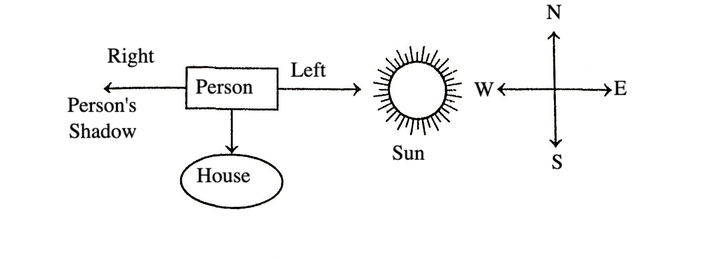Clearly, the person is walking towards the South direction.

Type #2: Based on Displacement

In this type of questions, we have to find out the final distance between the starting and the final points or distance between two points/persons/things.

Example 1:  Vijay travelled 12 km Southward, then turned right and travelled 10 km, then turned right and travelled 12 km. How far was Vijay from the starting point?

A. 22 km     B. 44 km     C. 12 km    D. 10 km

Solution:  (D) According to the question, direction diagram of Vijay is a given below.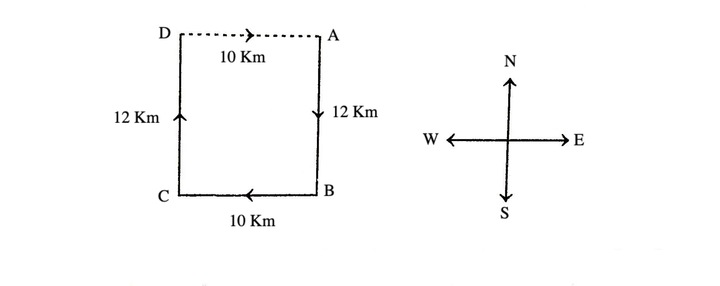Let point A be the starting point and D be the final point of travelling of Vijay.

Now, Vijay's distance from starting point
= AD = BC = 10 km

Example 2:  A person starts from a point A and travels 3 km Eastwards to B and then turns left and travels thrice that distance to reach C. He again turns left and travels five times the distance he covered between A and B and reaches his destination D. The shortest distance between the starting point and the destination is

A. 12 km              B. 15 km
C. 16 km              D. 18 km

Solution:  (B) According to the question, the movements of the person are as given below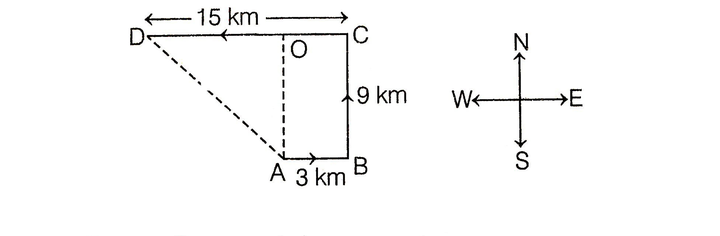According to the question,

BC = (3 x 3) = 9 km

CD = (3 x 5) = 15 kmOD = (15 - 3) = 12 km

Now, required distance,
= 122 + 92 = 144 + 81
= 225 = 15 km

Type #3: Based on Direction and Displacement

In this type of questions, we have to determine both the distance and direction.

Example 1:  Shyam, goes 5 km in the North from his school. Now, turning to the left, he goes to 10 km and again turns to left and goes to 5 km. How far he is from his school and in which direction?

A. 10 km, South from school

B. 10 km, North from school

C. 10km, West from school

D. 10 km, East from school

Solution:  (C) According to the question, the movements of Shyam are given below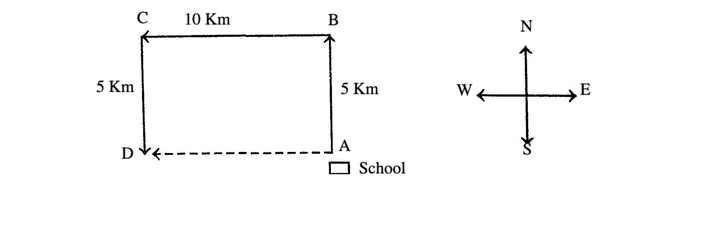Let point A be the starting point, i.e. school of Shyam and point D be the final point.

From figure, AB = Cd = 5 km
and Ad = BC = 10 km

So, Shyam is 10 km far away from his school and in West direction from school.

Example 2:  x and Y start from the same point X walks 40 m North, then turns West and walks 80 m, then turns to his right and walks 50 m. At the same time, Y walks 90 m North. Where is Y now with respect to the position of X?

A. Y is 30 m to the East of X

B. Y is 80 m to the West of X

C. Y is 30 m to the west of X

D. Y is 80 m to the East of X

Solution:  (D) According to the question, diagram is as given below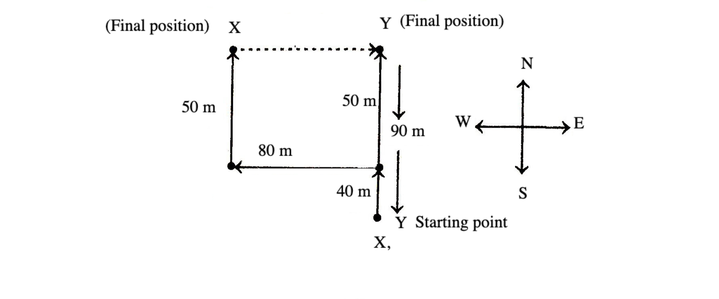it is clear from diagram that, Y is 80 m to the East of X.

LearnFrenzy provides you lots of fully solved Direction Sense Test Questions and Answers with explanation.